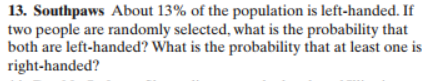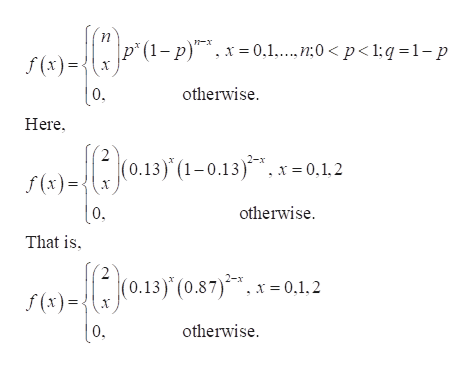# 13. Southpaws About 13% of the population is left-handed. Iftwo people are randomly selected, what is the probability thatboth are left-handed? What is the probability that at least one isright-handed?

Question
81 viewshelp_outlineImage Transcriptionclose13. Southpaws About 13% of the population is left-handed. If two people are randomly selected, what is the probability that both are left-handed? What is the probability that at least one is right-handed? fullscreen
check_circle

star
star
star
star
star
1 Rating
Step 1

Identifying the distribution:

Consider the event X that a people having left hand as a “success”.

There are 2 people. Each question can be considered as a trial. So, there are n = 2 trials.

Now, the people are randomly selected. Moreover, one people is independent of another. So, the 2 trials are independent of one another.

Here, the probability of getting a “Success” is 0.13.

The probability of a success is p = 0.13, which is constant for all trials.

Consider X as the number of successes (number of people having left-handed) out of the n = 2 trials.

Thus, X has a Binomial distribution with parameters n = 2 and p = 0.13. Since X is a discrete random variable, X has a Binomial pmf (probability mass function).help_outlineImage Transcriptionclosep" (1-р)", х -0.1....»:0 < p<1:q-1-р n-x f (x) |0. Here otherwise 2 f (x)= x(0.13) (1-0.13)*, x=0,1.2 otherwise [0. That is 2 (0.13) (0.87).x0.1,2 f (x) otherwise |0, fullscreen
Step 2

The required probability is P (Both are left-handed). The required probability is obtained as shown below:

Step 3

Thus, the probability P (Both are ...

### Want to see the full answer?

See Solution

#### Want to see this answer and more?

Solutions are written by subject experts who are available 24/7. Questions are typically answered within 1 hour.*

See Solution
*Response times may vary by subject and question.
Tagged in

### Hypothesis Testing